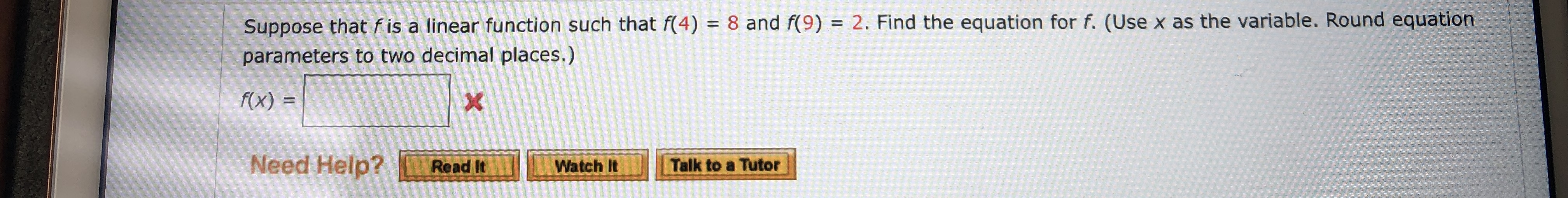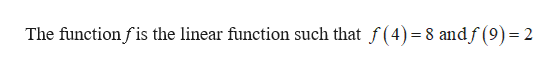Suppose that f is a linear function such that f(4) = 8 and f(9) = 2. Find the equation for f. (Use x as the variable. Round equationparameters to two decimal places.)f(x) =XNeed Help?Talk to a TutorRead ItWatch It

Question

What are the blanks?help_outlineImage TranscriptioncloseSuppose that f is a linear function such that f(4) = 8 and f(9) = 2. Find the equation for f. (Use x as the variable. Round equation parameters to two decimal places.) f(x) = X Need Help? Talk to a Tutor Read It Watch It fullscreen
Step 1

Given informa...help_outlineImage TranscriptioncloseThe function fis the linear function such that f(4)=8 andf (9)= 2 fullscreen

Want to see the full answer?

See Solution

Want to see this answer and more?

Our solutions are written by experts, many with advanced degrees, and available 24/7

See Solution
Tagged in

Other